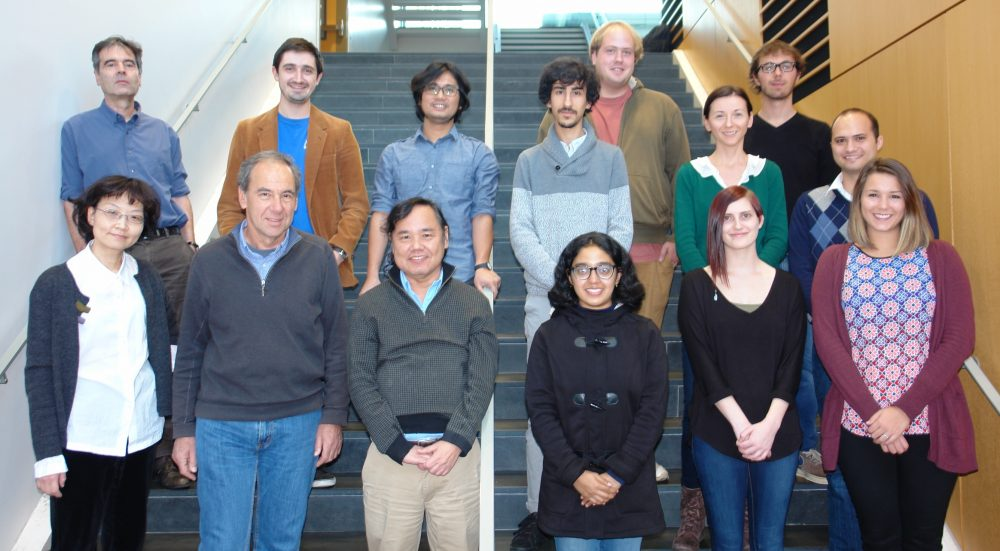# WelcomeLinear functional analysis was developed mostly in the first half of the 20th century. Around the 1960s several extensions of linear objects were proposed, for instance the theory of nonexpansive operators as an extension of unitary operators, the theory of monotone operators as an extension of the theory of positive operators, or convex analysis as an extension of classical linear sets and functionals. Such extensions of structured theories outside of linear functional analysis form what is called nonlinear analysis.

Our research group investigates various aspects of the theory of nonlinear analysis and of its applications to fields such as ordinary and partial differential equations, evolution equations, probability and stochastic processes, optimization, game theory, fixed point theory, and monotone operator theory. We also study applications in concrete areas such as inverse problems, biomathematics, data science, machine learning, finance, image recovery, fluid mechanics, and signal processing.